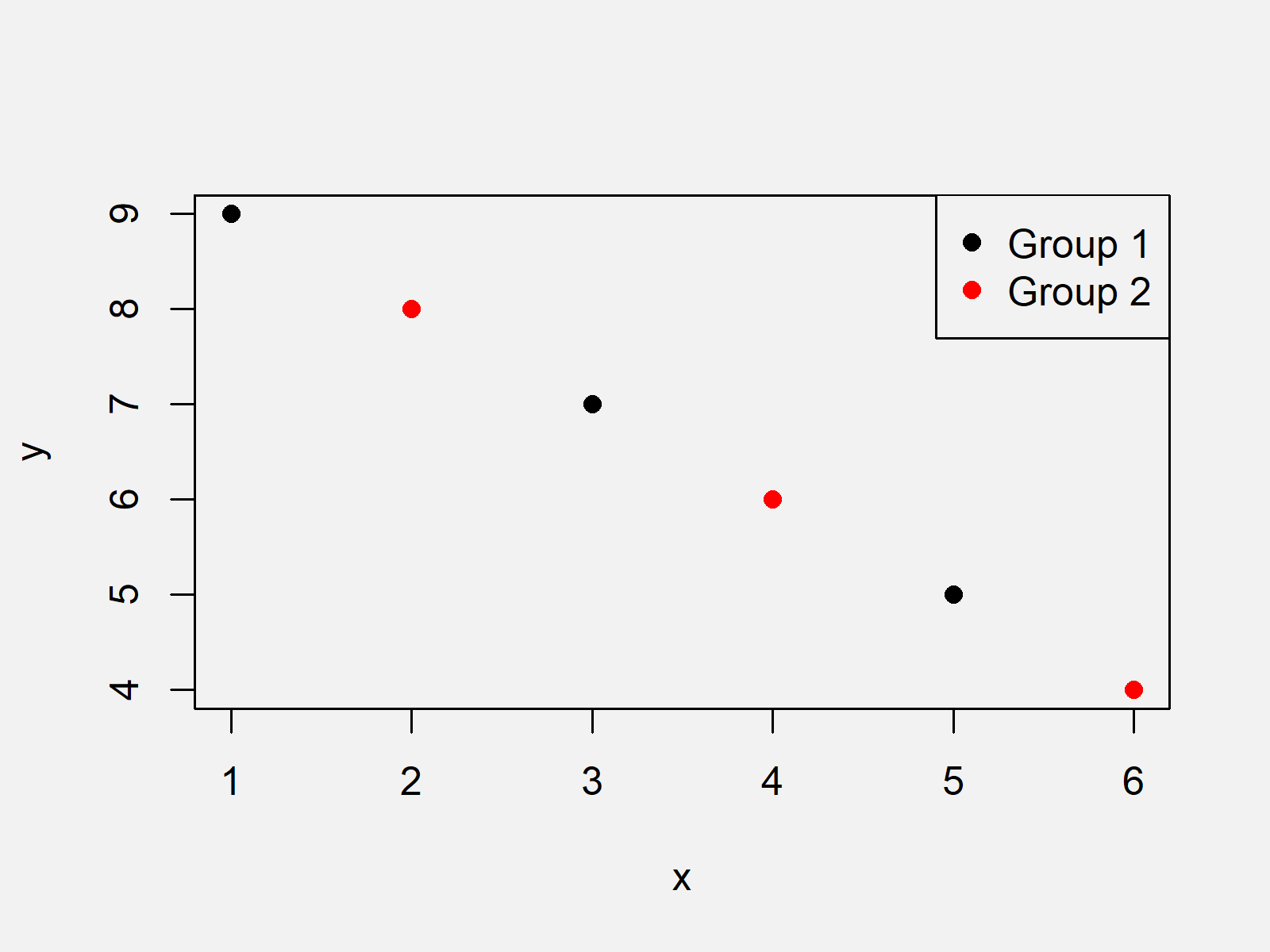# Change Legend Size in Base R Plot (2 Examples)

In this R tutorial you’ll learn how to adjust the size of a plot legend.

The article will contain the following topics:

Let’s dive right into the examples:

## Introduction of Example Data

As a first step, we’ll need to create some data that we can use in the examples below:

```x <- 1:6                  # Create example data
y <- 9:4
group <- rep(1:2, 3)```

Now, we can draw these data and a default legend as shown below:

```plot(x, y,                # Draw plot in Base R
col = group,
pch = 16)
legend("topright",        # Add legend to plot
legend = c("Group 1", "Group 2"),
col = 1:2,
pch = 16)```The output of the previous R syntax is shown in Figure 1 – A Base R scatterplot containing a legend with default size.

Let’s make this legend smaller and larger!

## Example 1: Decrease Legend Size Using cex Argument

In Example 1, I’ll explain how to use the cex argument of the legend function to decrease the legend size of a Base R plot. Have a look at the following R code:

```plot(x, y,                # Draw plot in Base R
col = group,
pch = 16)
legend("topright",        # Add legend to plot
legend = c("Group 1", "Group 2"),
col = 1:2,
pch = 16,
cex = 0.5)         # Decrease legend size```As shown in Figure 2, the previous code created a graph with smaller legend.

Note that we only had to specify the cex argument of the legend function. Besides that, everything was kept the same as in the default plot we have created before.

## Example 2: Increase Legend Size Using cex Argument

The R programming code below shows how to create a large legend using the cex argument.

In contrast to the previous example, we have to specify a value larger than 1 for the cex argument to increase the size of our legend.

```plot(x, y,                # Draw plot in Base R
col = group,
pch = 16)
legend("topright",        # Add legend to plot
legend = c("Group 1", "Group 2"),
col = 1:2,
pch = 16,
cex = 2.5)         # Increase legend size```The output of the previous code is shown in Figure 3 – A plot with larger legend size.

## Video & Further Resources

Please accept YouTube cookies to play this video. By accepting you will be accessing content from YouTube, a service provided by an external third party.If you accept this notice, your choice will be saved and the page will refresh.

In addition, you might read the other articles of my website. I have published numerous tutorials on topics such as graphics in R, ggplot2, and plot legends.

At this point you should have learned how to increase or decrease the legend size in a graphic in R. In case you have any additional questions, don’t hesitate to let me know in the comments.

Subscribe to the Statistics Globe Newsletter